# Rotary Encoder for Controlling Stepper and Servo Motors.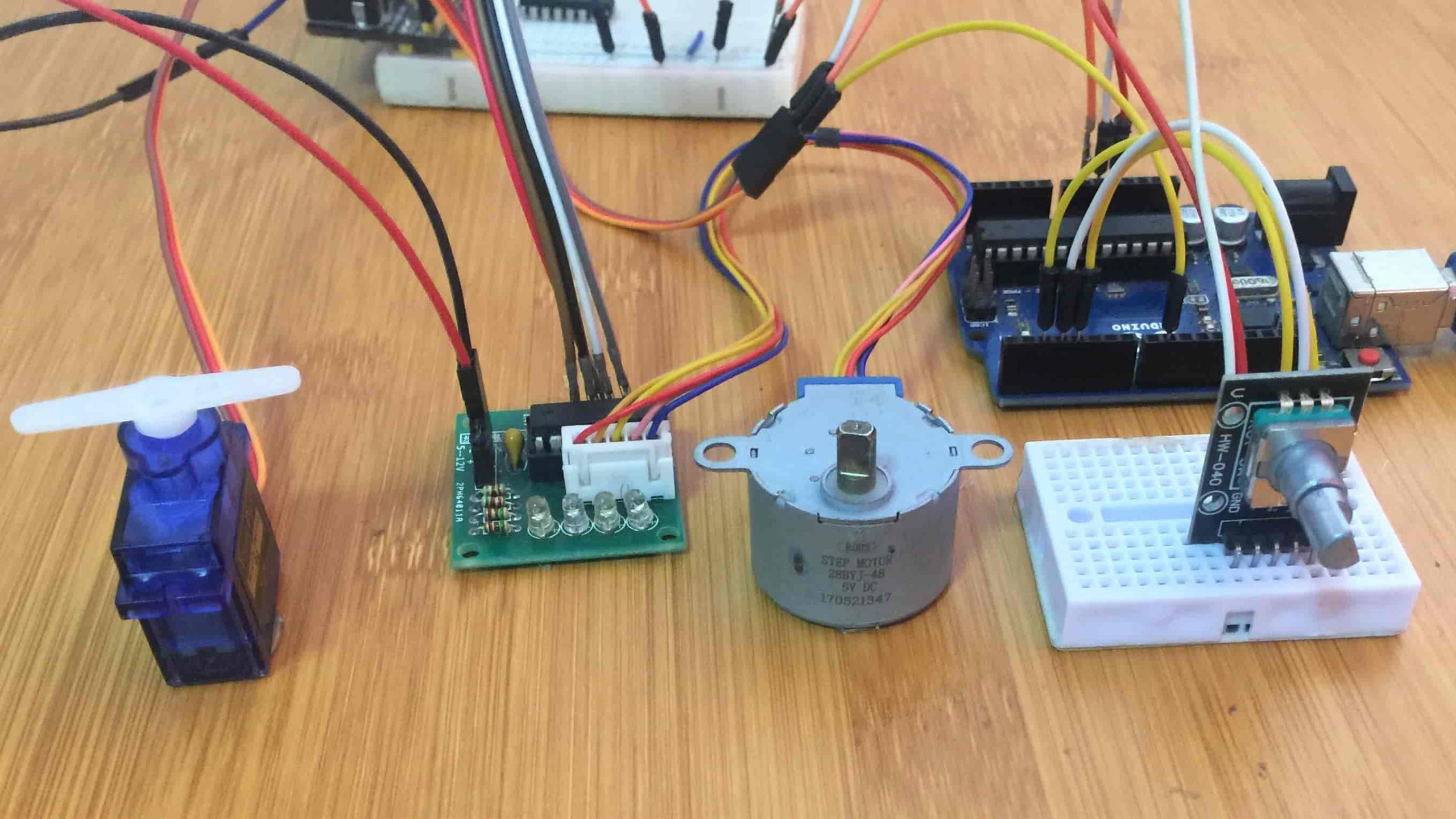Table of Contents

A rotary encoder is an electro-mechanical device that can convert the angular position of a shaft to either an analog or digital output signals that can be used to determine what direction the shaft is being rotated.
Rotary encoders come in various types but we are mainly looking at the incremental encoder which is also known as a quadrature rotary encoder due to how the device works.

## How a rotary encoder works.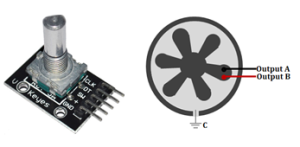The encoder has a knob attached to a disk with evenly spaced contact zones that are connected to the common pin C and two other separate contact pins A and B which are labelled CLK and DT respectively.
When the knob is turned, the disk will start rotating step by step and the pins A and B will start making contact with the common ground pin C. This generates two square wave signals that are interpreted to determine the direction of rotation. This is illustrated in the diagram below.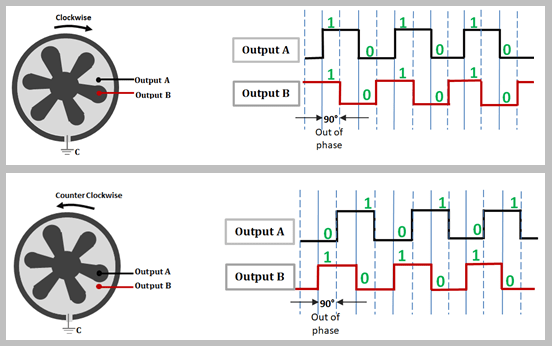If the knob is turned clockwise, output A connects to the ground first followed by the B pin. For the counter clockwise direction the movement is in the opposite direction. In each case the generated square waves are read by the microcontroller.
The two output signals are at 90 degrees out of phase from each other. The direction of rotation can be determined by the state of the signals. For example in the above case when both signals are the same, the rotation is clockwise and if the signals are opposite, the rotation is counter clockwise.

## Connecting the rotary encoder to Arduino.

Connecting the Rotary Encoder to Arduino is simple. Connect the + pin on the module to 5V on the Arduino and GND pin to ground. The CLK, DT and SW pins are connected to any of digital pins of the Arduino.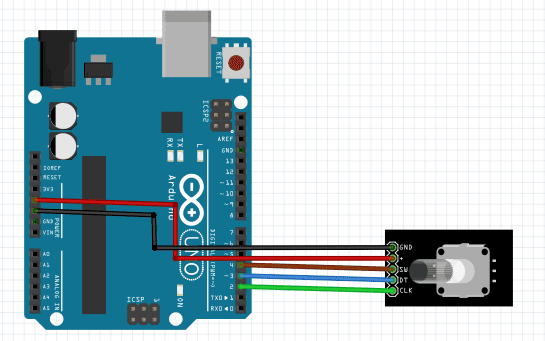Let’s first take an example where we can read the direction of rotation and the number of steps of rotation and display them on a serial monitor.

### Code

```// Rotary Encoder Inputs
#define CLK 2
#define DT 3
#define SW 4

int counter = 0;
int currentStateCLK;
int lastStateCLK;
String currentDir ="";
unsigned long lastButtonPress = 0;

void setup() {
pinMode(CLK,INPUT);
pinMode(DT,INPUT);
pinMode(SW, INPUT_PULLUP);
// Setup Serial Monitor
Serial.begin(9600);
// Read the initial state of CLK
lastStateCLK = digitalRead(CLK);
}

void loop() {

// Read the current state of CLK
currentStateCLK = digitalRead(CLK);
// If last and current state of CLK are different, then pulse occurred
// React to only 1 state change to avoid double count
if (currentStateCLK != lastStateCLK  && currentStateCLK == 1){
// If the DT state is different than the CLK state then
// the encoder is rotating CCW so decrement
if (digitalRead(DT) != currentStateCLK) {
counter --;
currentDir ="CCW";
} else {
// Encoder is rotating CW so increment
counter ++;
currentDir ="CW";
}
Serial.print("Direction: ");
Serial.print(currentDir);
Serial.print(" | Counter: ");
Serial.println(counter);
}
// Remember last CLK state
lastStateCLK = currentStateCLK;
// Read the button state
int btnState = digitalRead(SW);
//If we detect LOW signal, button is pressed
if (btnState == LOW) {
//if 50ms have passed since last LOW pulse, it means that the
//button has been pressed, released and pressed again
if (millis() - lastButtonPress > 50) {
Serial.println("Button pressed!");
}
// Remember last button press event
lastButtonPress = millis();
}
// Put in a slight delay to help debounce the reading
delay(1);
}
```
When the above code is uploaded to the Arduino, we can be able to rotate the knob of the encoder and observe the values on the serial monitor as shown below.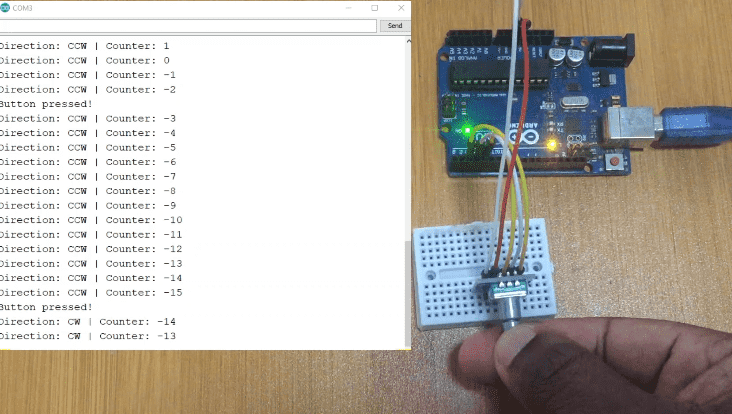## Controlling motors using a rotary encoder.

Since rotary encoders are very good at measuring mechanical rotation they can easily be used to control rotation of motors.

### Stepper Motor control.

Before connecting the setup shown below you can make reference to my other tutorial on how to control the 28BYJ-48 stepper motor with the ULN2003 board and Arduino using the link:
• 28BYJ-48 Stepper motor Control with the ULN2003 Driver and Arduino.
•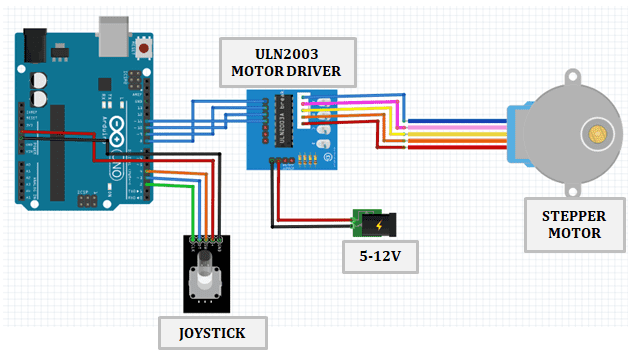I must emphasize that never power a motor directly from the Arduino power source because this will destroy the Arduino board.

#### Code for controlling stepper motor using rotary encoder.

```#include "Stepper.h"
#define STEPS  32   // Number of steps for one revolution of Internal shaft
// 2048 steps for one revolution of External shaft

volatile boolean TurnDetected;  // need volatile for Interrupts
volatile boolean rotationdirection;  // CW or CCW rotation

const int PinCLK=2;   // Generating interrupts using CLK signal
const int PinDT=3;    // Reading DT signal
const int PinSW=4;    // Reading Push Button switch

int RotaryPosition=0;    // To store Stepper Motor Position

int PrevPosition;     // Previous Rotary position Value to check accuracy
int StepsToTake;      // How much to move Stepper

// Setup of proper sequencing for Motor Driver Pins
// In1, In2, In3, In4 in the sequence 1-3-2-4
Stepper small_stepper(STEPS, 8, 10, 9, 11);

// Interrupt routine runs if CLK goes from HIGH to LOW
void isr ()  {
delay(4);  // delay for Debouncing
if (digitalRead(PinCLK))
rotationdirection= digitalRead(PinDT);
else
rotationdirection= !digitalRead(PinDT);
TurnDetected = true;
}

void setup ()  {

pinMode(PinCLK,INPUT);
pinMode(PinDT,INPUT);
pinMode(PinSW,INPUT);
digitalWrite(PinSW, HIGH); // Pull-Up resistor for switch
attachInterrupt (0,isr,FALLING); // interrupt 0 always connected to pin 2 on Arduino UNO
}

void loop ()  {
small_stepper.setSpeed(600); //Max seems to be 700
if (!(digitalRead(PinSW))) {   // check if button is pressed
if (RotaryPosition == 0) {  // check if button was already pressed
} else {
small_stepper.step(-(RotaryPosition*50));
RotaryPosition=0; // Reset position to ZERO
}
}

// Runs if rotation was detected
if (TurnDetected)  {
PrevPosition = RotaryPosition; // Save previous position in variable
if (rotationdirection) {
RotaryPosition=RotaryPosition-1;} // decrase Position by 1
else {
RotaryPosition=RotaryPosition+1;} // increase Position by 1

TurnDetected = false;  // do NOT repeat IF loop until new rotation detected

// Which direction to move Stepper motor
if ((PrevPosition + 1) == RotaryPosition) { // Move motor CW
StepsToTake=50;
small_stepper.step(StepsToTake);
}

if ((RotaryPosition + 1) == PrevPosition) { // Move motor CCW
StepsToTake=-50;
small_stepper.step(StepsToTake);
}
}
}
```

### Servo Motor control.

In case you need reference on how to interface a servo motor with Arduino you can check my previous tutorial from the link:
• How to control a Servo motor with Arduino.
•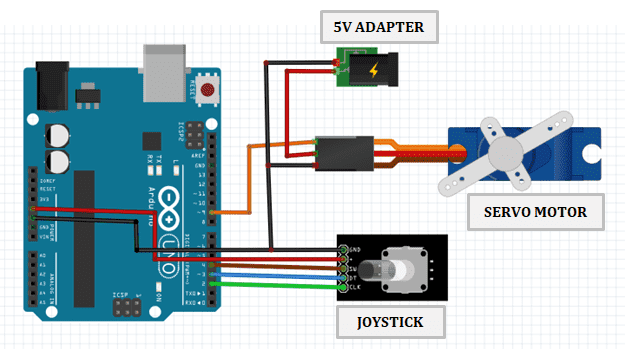#### Code for controlling servo motor using rotary encoder.

```#include <Servo.h>

// Rotary Encoder Inputs
#define CLK 2
#define DT 3

Servo servo;
int counter = 0;
int currentStateCLK;
int lastStateCLK;

void setup() {

// Set encoder pins as inputs
pinMode(CLK,INPUT);
pinMode(DT,INPUT);

// Setup Serial Monitor
Serial.begin(9600);

// Attach servo on pin 9 to the servo object
servo.attach(9);
servo.write(counter);

// Read the initial state of CLK
lastStateCLK = digitalRead(CLK);
}

void loop() {

// Read the current state of CLK
currentStateCLK = digitalRead(CLK);

// If last and current state of CLK are different, then pulse occurred
// React to only 1 state change to avoid double count
if (currentStateCLK != lastStateCLK  && currentStateCLK == 1){

// If the DT state is different than the CLK state then
// the encoder is rotating CCW so decrement
if (digitalRead(DT) != currentStateCLK) {
counter --;
if (counter<0)
counter=0;
} else {
// Encoder is rotating CW so increment
counter ++;
if (counter>179)
counter=179;
}
// Move the servo
servo.write(counter);
Serial.print("Position: ");
Serial.println(counter);
}

// Remember last CLK state
lastStateCLK = currentStateCLK;
}
```
Back to top